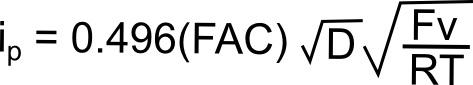# Diffusion Coefficient

Honestly, I don't have a definition of diffusion coefficients that's any better than wikipedia, but that's fine because I can count on one hand the number of times I've needed to find it. If you have an analyte that undergoes a reversible electron transfer during a CV, you can determine its diffusion coefficient the peak current (ip) of the reversible wave using the following equation#### hover over pic for description

where D is the diffusion coefficient (cm2/s), F is Faraday's constant (96,485 C mol–1), A is the area of the electrode (cm2), C is the concentration of the analyte in the bulk solution (mol cm–3), v is the scan rate (V/s), T is the temperature (in Kelvin), R is the gas constant (8.315 J K–1 mol–1).